﻿ Mass particle inflow into cyclone flow - XSim

# Mass particle inflow into cyclone flow

Update: March 16, 2018
OpenFOAM 4.x

## Case directory

\$FOAM_TUTORIALS/lagrangian/MPPICFoam/cyclone

## Summary

Air flows into the cylindrical container at (-10, 0, 0) m/s through the inlet (red color in the figure below) and out through the outlet (blue color in the figure below). The total length of the calculation is 7 seconds, with particles flowing in through the inlet for 4 seconds from at time 1 second.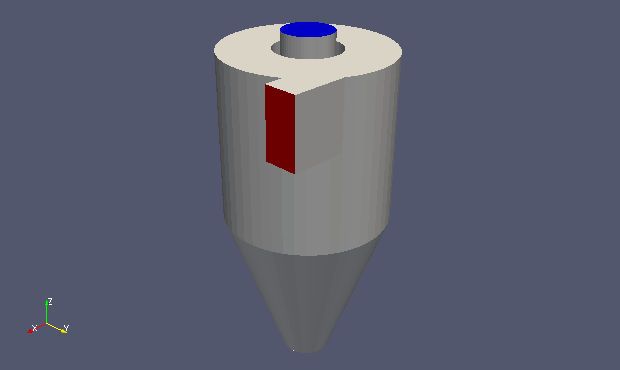Model geometry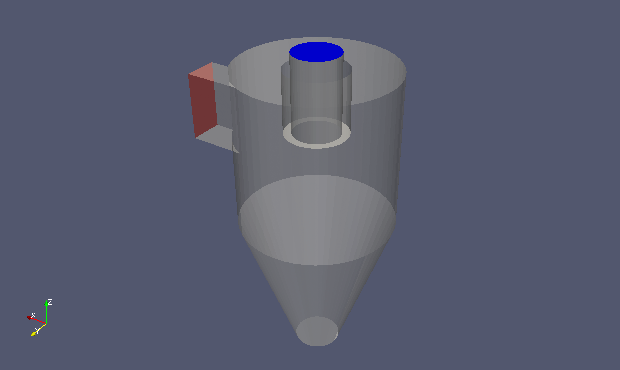Model geometry (semi-transparent display)

The physical properties of discrete particles are defined in the file "kinematicCloudProperties" in the directory "constant". The position of particle inflow, inflow start time, inflow duration, velocity, size distribution, etc. are defined in "injectionModels" of "subModels" as follows.

```injectionModels
{
model1
{
type patchInjection;
massTotal 40;
SOI 1;
parcelBasisType mass;
patchName inlet;
duration 4;
parcelsPerSecond 100644;
U0 (-10 0 0);
flowRateProfile constant 1;
sizeDistribution
{
type normal;
normalDistribution
{
expectation 100e-6;
variance 25e-6;
minValue 20e-6;
maxValue 180e-6;
}
}
}
}
```

The meshes are as follows, and the number of mesh is 34240.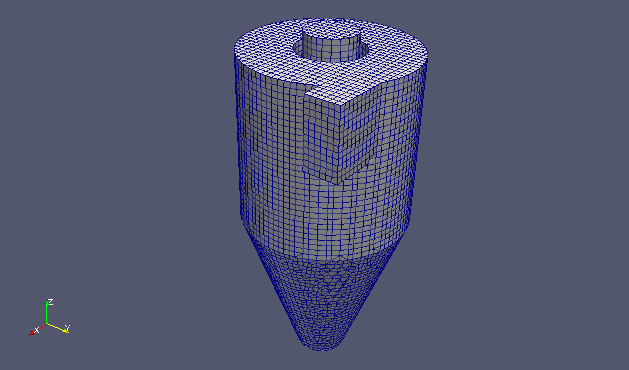Meshes

The calculation result is as follows.

Discrete particles's motion (After the start of particle inflow, the color represents velocity)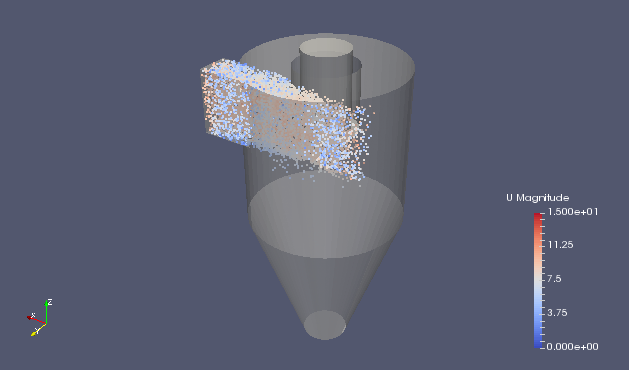Discrete particles and the velocity at 1.1 sec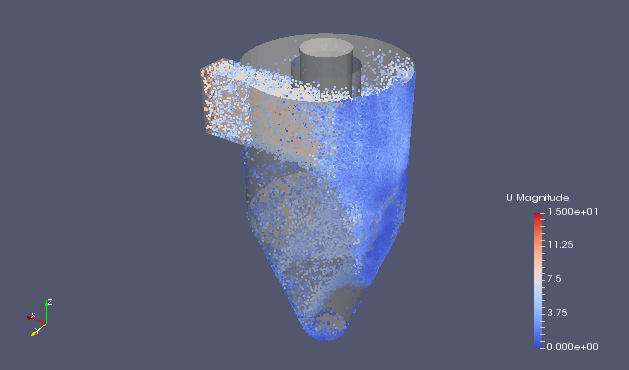Discrete particles and the velocity at 2 sec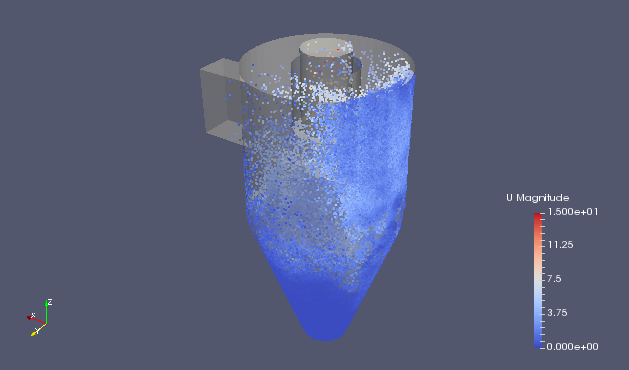Discrete particles and the velocity at 5.1 sec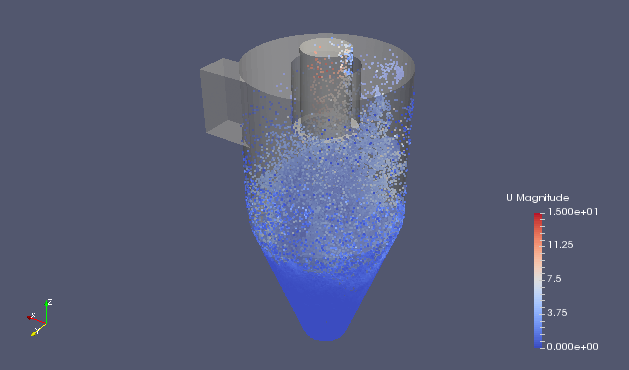Discrete particles and the velocity at 6 sec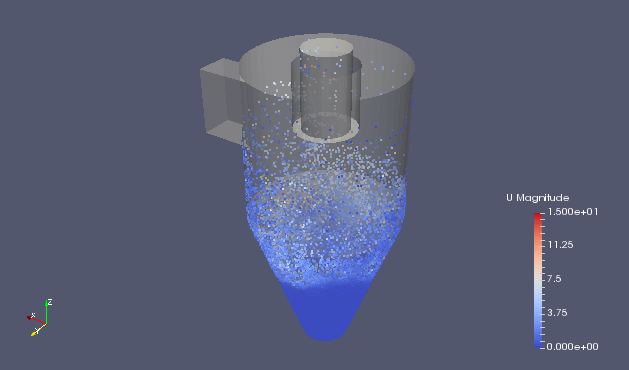Discrete particles and the velocity at 7 sec

## Commands

cp -r \$FOAM_TUTORIALS/lagrangian/MPPICFoam/cyclone cyclone
cd cyclone

cp \$FOAM_TUTORIALS/resources/geometry/cyclone.stl.gz constant/triSurface

blockMesh
snappyHexMesh -overwrite
decomposePar
mpirun -np 12 MPPICFoam -parallel
reconstructParMesh
reconstructPar

paraFoam

## Calculation time

13 hours 58 minutes 33.1 seconds *12 parallel, Inter(R) Core(TM) i7-2600 CPU @ 3.40GHz 3.40GHz